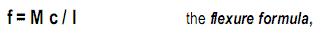## Wednesday, June 4, 2014

### BEAMS FLEXURE FORMULA

The flexure formula gives the internal bending stress caused by an external moment on a beam or other bending member of homogeneous material.  It is derived here for a rectangular beam but is valid for any shape.

1  Unloaded beam with hatched square
2  Beam subject to bending with hatched square deformed
3  Stress diagram of deformed beam subject to bending

Referring to the diagram, a beam subject to positive bending assumes a concave curvature (circular under pure bending).  As  illustrated by the hatched square, the top shortens and the bottom elongates, causing compressive stress on the top and tensile stress on the bottom.  Assuming stress varies linearly with strain, stress distribution over the beam depth is proportional to strain deformation.  Thus stress varies linearly over the depth of the beam and is zero at the neutral axis (NA).  The bending stress fy at any  distance y from the neutral axis is found, considering similar triangles, namely fy relates  to y as f relates to c; f is the maximum bending stress at top or bottom and c the distance  from the Neutral Axis, namely fy / y = f / c. Solving for fy yields

fy = y f / c

To satisfy equilibrium, the beam requires an internal resisting moment that is equal and  opposite to the external bending moment.  The internal resisting moment is the sum of all partial forces F rotating around the neutral axis with a lever arm of length y to balance the external moment.  Each partial force F is the productof stress fy and the partial area a on which it acts, F = a fy.  Substituting fy = y f / c, defined above, yields F = a y f / c.  Since the internal resisting moment M is the sum of all forces F times their lever arm y to the  neutral axis, M = F y = (f/c) Σ y y a = (f/c) Σ y^2 a, or M = I f/c, where the term Σy^2 a is defined as  moment of inertia (I =  Σy^2 a) for convenience.  In formal calculus the summation of area  a is replaced by integration of the differential area  da, an infinitely small area:
The internal resisting moment equation M = I f/c solved for stress f yieldswhich gives the bending stress f at any distance c from the neutral axis.  A simpler form  is used to compute the  maximum fiber stress as derived before.  Assuming c as  maximum fiber distance from the neutral axis yields:
Both the moment of inertia I and section modulus S define the strength of a cross-section regarding its geometric form.